Courses

# Joint Probability Distributions Mathematics Notes | EduRev

## Mathematics : Joint Probability Distributions Mathematics Notes | EduRev

The document Joint Probability Distributions Mathematics Notes | EduRev is a part of the Mathematics Course Additional Topics for IIT JAM Mathematics.
All you need of Mathematics at this link: Mathematics

Joint Probability Distributions
In the section on probability distributions, we looked at discrete and continuous distributions but we only focused on single random variables. Probability distributions can, however, be applied to grouped random variables which gives rise to joint probability distributions. Here we're going to focus on 2-dimensional distributions (i.e. only two random variables) but higher dimensions (more than two variables) are also possible.

Since all random variables are divided into discrete and continuous random variables, we have end up having both discrete and continuous joint probability distributions. These distributions are not so different from the one variable distributions we just looked at but understanding some concepts might require one to have knowledge of multivariable calculus at the back of their mind.

Essentially, joint probability distributions describe situations where by both outcomes represented by random variables occur. While we only X to represent the random variable, we now have X and Y as the pair of random variables.
Joint probability distributions are defined in the form below:
f(x, y) = P(X = x, Y = y)
where by the above represents the probability that events x and y occur at the same time.
The Cumulative Distribution Function (CDF) for a joint probability distribution is given by: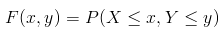Discrete Joint Probability Distributions
Discrete random variables when paired give rise to discrete joint probability distributions. As with single random variable discrete probability distribution, a discrete joint probability distribution can be tabulated as in the example below.
The table below represents the joint probability distribution obtained for the outcomes when a die is flipped and a coin is tossed.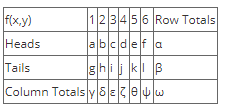In the table above, x = 1, 2, 3, 4, 5, 6 as outcomes when the die is tossed while y = Heads, Tails are outcomes when the coin is flipped. The letters a through l represent the joint probabilities of the different events formed from the combinations of x and y while the Greek letters represent the totals and ω should equal to 1. The row sums and column sums are referred to as the marginal probability distribution functions (PDF).
We shall see in a moment how to obtain the different probabilities but first let us define the probability mass function for a joint discrete probability distribution.
The probability function, also known as the probability mass function for a joint probability distribution f(x,y) is defined such that:

• f(x,y) ≥ 0 for all (x,y)
Which means that the joint probability should always greater or equal to zero as dictated by the fundamental rule of probability.
• ∑x ∑y f(x,y) = 1
Which means that the sum of all the joint probabilities should equal to one for a given sample space.
• f(x,y) = P(X =x, Y = y)
The mass probability function f(x,y) can be calculated in a number of different ways depend on the relationship between the random variables X and Y.

As we saw in the section on probability concepts, these two variables can be either independent or dependent.
If X and Y are Independent: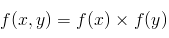In the example we gave above, flipping a coin and tossing a die are independent random variables, the outcome from one event does not in any way affect the outcome in the other events. Assuming that the coin and die were both fair, the probabilities given by a through l can be obtained by multiplying the probabilities of the different x and y combinations.
For example: P(X = 2, Y = Tails) is given by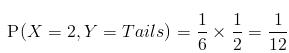Since we claimed that the coin and the die are fair, the probabilities a through l should be the same.
The marginal PDF's, represented by the Greek letters should be the probabilities you expect when you obtain each of the outcomes.
For example: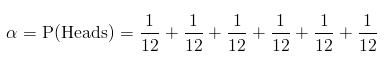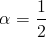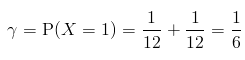The table thus becomes: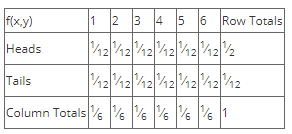If X and Y are Dependent:
If X and Y are dependent variables, their joint probabilities are calculated using their different relationships as in the example below.
Given a bag containing 3 black balls, 2 blue balls and 3 green balls, a random sample of 4 balls is selected. Given that X is the number of black balls and Y is the number of blue balls, find the joint probability distribution of X and Y.
Solution:
The random variables X and Y are dependent since they are picked from the same sample space such that if any one of them is picked, the probability of picking the other is affected. So we solve this problem by using combinations.
We've been told that there are 4 possible outcomes of X i.e {0,1,2,3} where by you can pick none, one, two or three black balls; and similarly for Y there are 3 possible outcomes {0,1,2} i.e. none, one or two blue balls.
The joint probability distribution is given by the table below: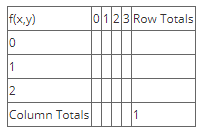To fill out the table, we need to calculate the different entries. We know the total number of black balls to be 3, the total number of blue balls to be 2, the total sample need to be 4 and the total number of balls in the bag to be 3+2+3 = 8.
We find the joint probability mass function f(x,y) using combinations as: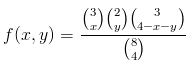What the above represents are the different number of ways we can pick each of the required balls. We substitute for the different values of x (0,1,2,3) and y (0,1,2) and solve i.e.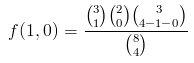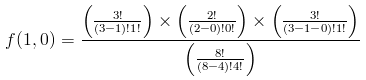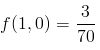f(0,0) is a special case. We don't calculate this and we outright claim that the probability of obtaining zero black balls and zero blue balls is zero. This is because of the size of the entire population relative to the sample space. We need 4 balls from a bag of 8 balls, in order not to pick black nor blue balls, we would need there to be at least 4 green balls. But we only have 3 green balls so we know that as a rule we must have at least either one black or blue ball in the sample.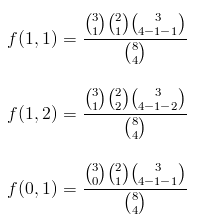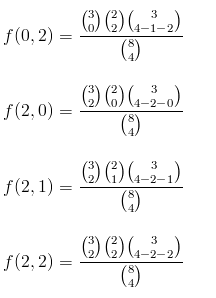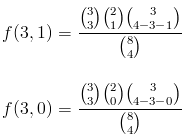f(3,2) doesn't exist since we only need 4 balls.
From the above, we obtain the joint probability distribution as: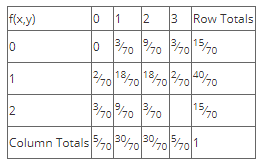Continuous Joint Probability Distribution
Continuous Joint Probability Distributions arise from groups of continuous random variables.
Continuous joint probability distributions are characterized by the Joint Density Function, which is similar to that of a single variable case, except that this is in two dimensions.
The joint density function f(x,y) is characterized by the following:

• f(x,y) ≥ 0, for all (x,y)
• f(x,y) dx dy = 1
• For any region A lying in the xy plane,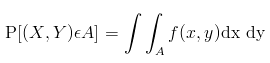The marginal probability density functions are given by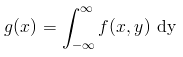whereby the above is the probability distribution of random variable X alone.
The probability distribution of the random variable Y alone, known as its marginal PDF is given by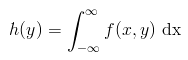Example:
A certain farm produces two kinds of eggs on any given day; organic and non-organic. Let these two kinds of eggs be represented by the random variables X and Y respectively. Given that the joint probability density function of these variables is given by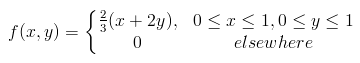(a) Find the marginal PDF of X
(b) Find the marginal PDF of Y
(c) Find the P(X ≤ 1⁄2, Y ≤ 1⁄2)
Solution:
(a) The marginal PDF of X is given by g(x) where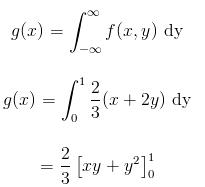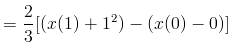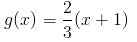(b) The marginal PDF of Y is given by h(y) where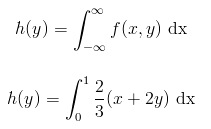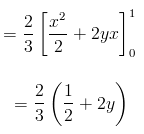(c) P(X ≤ 1⁄2, Y ≤ 1⁄2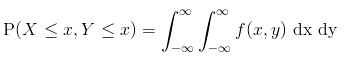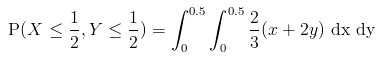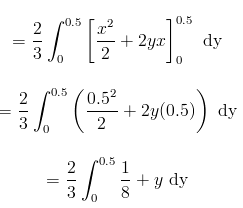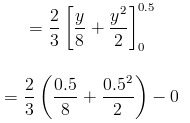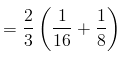= 1/8

Mixed Joint Probability Distribution
So far we've looked pairs of random variables where both variables are either discrete or continuous. A joint pair of random variables can also be composed of one discrete and one continuous random variable. This gives rise to what is known as a mixed joint probability distribution.
The density function for a mixed probability distribution is given by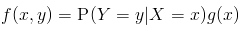where by X is a continuous random variable and Y is a discrete random variable, g(x) is the marginal pdf of X.
The cumulative distribution function is given by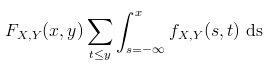Conditional Probability Distribution
Conditional Probability Distributions arise from joint probability distributions where by we need to know that probability of one event given that the other event has happened, and the random variables behind these events are joint.
Conditional probability distributions can be discrete or continuous, but the follow the same notation i.e.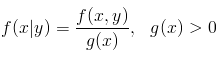where the above is the conditional probability of X given that Y = y.
The conditional probability of variable Y given that X = x is given by: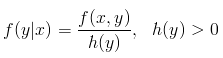The conditional probability distribution for a discrete set of random variables can be found from: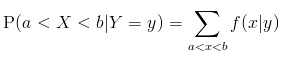where the above is the probability that X lies between a and b given that Y = y.
For a set of continuous random variables, the above probability is given as: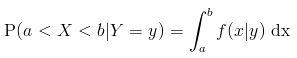Two random variables are said to be statistically independent if their conditional probability distribution is given by the following:
f(x, y) = g(x)h(y)
where g(x) is the marginal pdf of X and h(y) is the marginal pdf of Y.

Offer running on EduRev: Apply code STAYHOME200 to get INR 200 off on our premium plan EduRev Infinity!

40 docs

,

,

,

,

,

,

,

,

,

,

,

,

,

,

,

,

,

,

,

,

,

;